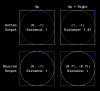# GMS 2Finding the Real Distance a Joystick is Pushed

#### Mookal

##### Member
I'm making a game where the character's speed is analog, based on how much the player pushes the left stick on a connected controller. I'd think finding the distance between the joystick's position and its origin would be simple:
GML:
``````var xpos = gamepad_axis_value(0, gp_axislh);
var dist = point_distance(0, 0, xpos, ypos);
return dist;``````
Unfortunately, this ends up causing a pretty major problem. While the area the joystick can move around in is a circle, that area is interpreted as a square.
So pushing up on the joystick would put its position at the top center of the square, but pushing both up and right on the joystick would put it in the top right of the square.
This means that, when the joystick is pushed towards a corner, the distance it's being pushed is overestimated.

I understand this concept is pretty abstract, so here's a diagram:Essentially, I want the interpreted distance from the origin to be the same no matter what direction the stick is held in, while also keeping analog movement. How can I achieve this?

#### PhallicSpleen

##### Member
First I would take the angle from the center to xpos/ypos, and figure out the distance to the edge of the square and the distance to the edge circle at that given angle. Then take the ratio of those numbers and apply it to the actual distance.

To figure out the distance to the edge of the square you could either use four collision line checks or MATHS https://math.stackexchange.com/ques...find-position-on-square-given-angle-at-center

#### Slyddar

##### Member
Try something like this :
Code:
``````if xpos != 0 or ypos != 0 {
var _dir = point_direction(0, 0, xpos, ypos);
var _f = 2; //speed factor
hsp = lengthdir_x(abs(xpos), _dir)*_f;
vsp = lengthdir_y(abs(ypos), _dir)*_f;
}``````
Then you can get dist with point_distance(0, 0, hsp, vsp)/_f if needed.

Last edited: